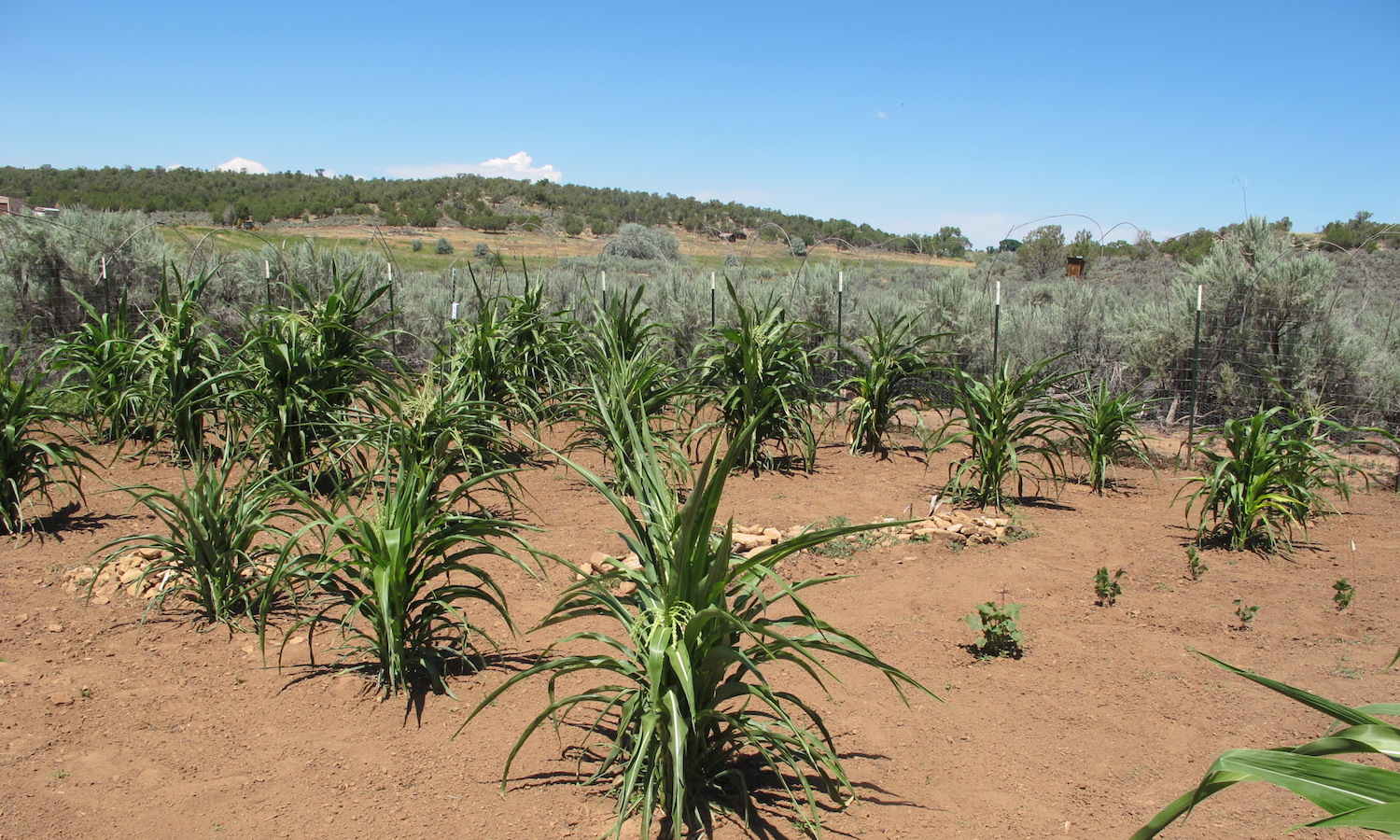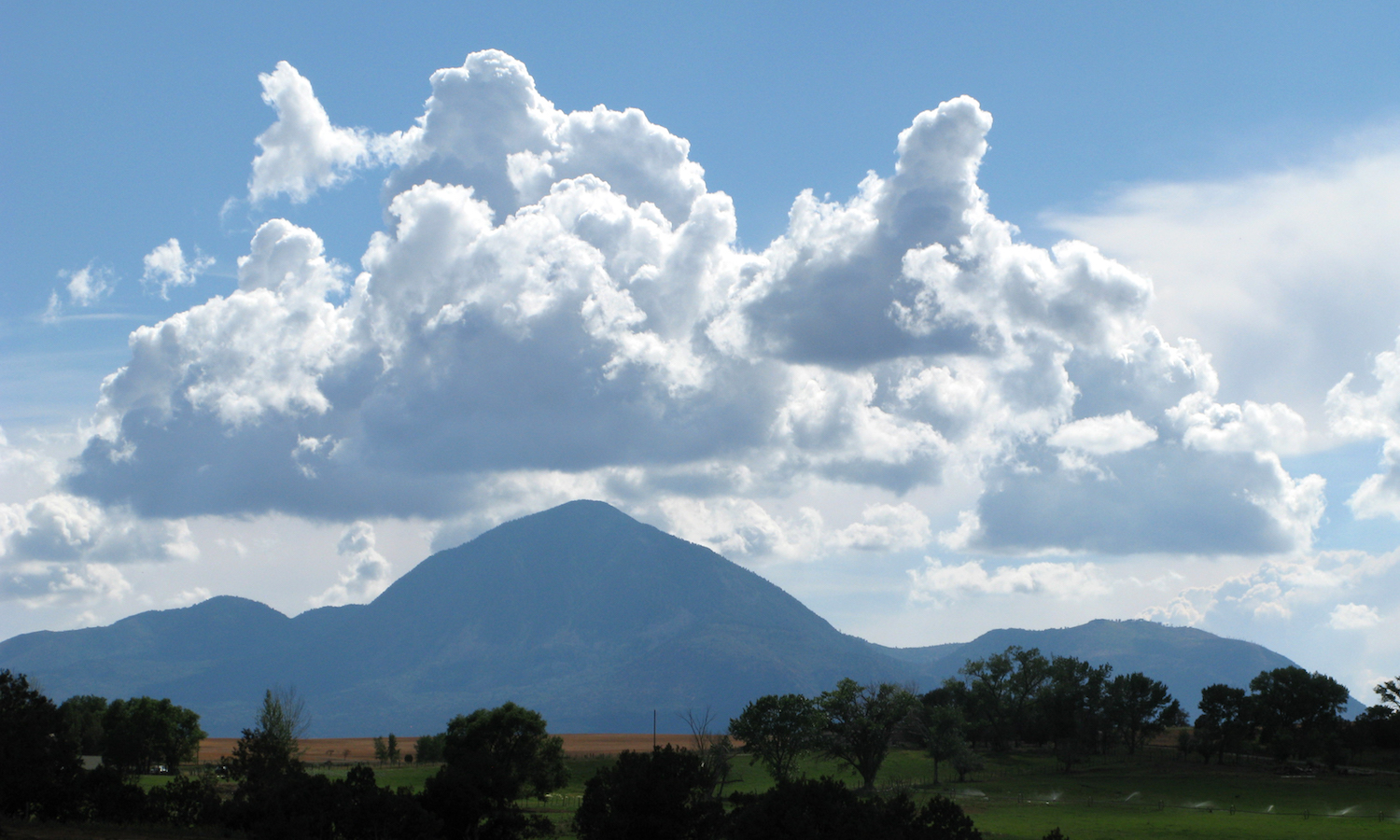## 4.2 Heat and sunlightThe Check Dam Garden on the Crow Canyon Archaeological Center campus.

All plants require thermal energy—in the form of heat and sunlight—to perform photosynthesis and flourish. Photosynthesis is a process used by plants to make a sugary food that fuels their growth. Plants absorb the energy of the sun through their leaves, combine it with water and carbon dioxide, and make a sugar called glucose. Fun Fact: The waste product that this process creates is oxygen! Farmers in ancient times adapted their corn to the amount of heat and sunlight available in the regions in which they lived, and farmers today do the same.

But how much heat does a plant need, and how do we know how much it gets? Plant researchers use a measure of accumulated heat called a growing degree day (GDD) to estimate the amount of heat that plants may use for photosynthesis.

The equation for daily GDD for maize is: $GDD=\frac{T_{MAX} + T_{MIN}}{2}-T_{BASE}$ where $$T_{MAX}$$ is the maximum daily temperature, $$T_{MIN}$$ is the minimum daily temperature and $$T_{BASE}$$ is the temperature below which plant growth ceases, which we take to be 10°C (~50ºF) for maize.

Here we use a series of corrections to the equation typically applied for calculating maize GDD, which down-corrects $$T_{MAX}$$ and $$T_{MIN}$$ to an upper threshold ($$T_{UT}$$, here 30°C or 86ºF) above which corn growth does not appreciably increase, and up-corrects $$T_{MAX}$$ and $$T_{MIN}$$ if they fall below $$T_{BASE}$$ (here 10°C or 50ºF). To summarize: $\text{if} \quad T_{MAX}>T_{UT}, \quad T_{MAX}=T_{UT}\\ \text{if} \quad T_{MIN}>T_{UT}, \quad T_{MIN}=T_{UT}\\ \text{if} \quad T_{MAX}<T_{BASE}, \quad T_{MAX}=T_{BASE}\\ \text{if} \quad T_{MIN}<T_{BASE}, \quad T_{MIN}=T_{BASE}$

Growing degree day amounts calculated in Celsius are different from those calculated in Fahrenheit. Growing degree days can be converted from Celsius heat units to Fahrenheit heat units by multiplying by a factor of 1.8. For example, 1000 Celsius GDDs are equal to 1800 Fahrenheit GDDs.

### Weather in CortezRain clouds gather over Ute Mountain in southwestern Colorado.

Plants combine water and nutrients from the soil with heat and energy from the Sun to flourish. Pueblo Farming Project researchers estimated the amount of water and accumulated heat available to the maize in the Pueblo Farming Project gardens using weather data gathered by the US National Oceanic and Atmospheric Administration (NOAA) as part of the US Historical Climatology Network (USHCN). Temperature and precipitation data from a weather station in Cortez were used to measure the response of the Pueblo Farming Project gardens to local weather; we also installed a weather station on Crow Canyon’s campus as well as temperature monitors in each garden. The graphs below show 2009 to 2018 data for the Pueblo Farming Project gardens. The top graph shows minimum and maximum daily temperatures and daily growing degree days, and the lower graph shows daily precipitation events. These graphs are interactive! Highlight a region of the graph in order to explore the weather. Hover over a graph to see the recorded weather at the Cortez weather station.